# Distances on Earth 1: The Cosine Formula

Many students study trigonometry, but few get to spherical trigonometry, the study of angles and distances on a sphere. This is particularly useful in dealing with measurements on the earth (though it is not a perfect sphere). In this series, we will derive and use three different formulas for the distance between points identified by their latitude and longitude: the cosine formula, the haversine formula, and a planar approximation. Of these, the first is easiest to explain, the second is most accurate, and the third is easiest to use, when appropriate.

## The cosine formula, first derivation

Using Longitude and Latitude to Determine Distance

I've been looking for the equation for finding the distance between two cities, given the latitude and longitude of both cities. For example: what is the formula one would use to find the distance between San Francisco (N37 37' 00" latitude, W122 22' 00" longitude) and Paris (N48 44' 00" latitude, E02 23' 00" longitude.)?

I'm trying to write a program in Visual Basic that will include this distance calculation in it.

Doctor Ken answered, deriving a formula using vectors:

This problem can be most easily solved by using spherical coordinates on the earth.  Have you dealt with those before?  Here's the transformation from spherical coordinates to normal rectangular coordinates, where a=latitude and b=longitude, and r is the radius of the earth:

x = r Cos[a] Cos[b]
y = r Cos[a] Sin[b]
z = r Sin[a]


Here is what we are talking about, where I’ve used α for the latitudes, β for the longitudes, and δ for the angle between the two vectors: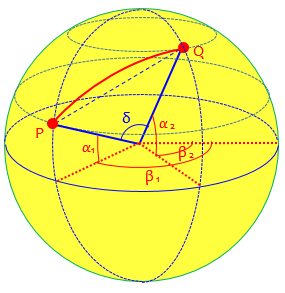The formulas for the x– and y-coordinates start with $$r\cos(\alpha)$$, which gives the radius of the parallel of latitude, and multiply by the cosine or sine of $$\beta$$ to find the horizontal distance in the appropriate direction. The formula for the z-coordinate is $$r\sin(\alpha)$$, which gives the vertical distance to the latitude line.

As we’ll see later, latitude is not quite the same as the angle we normally use in spherical coordinates mathematically.

Then we'll use the following property of the dot product (notated [p,q]):

[p,q] = Length[p] * Length[q] * Cos[angle between p & q]

He is using an alternate notation for the dot product, normally written as $$\mathbf{p}\cdot \mathbf{q}$$, to avoid confusion when typing. Our dot product between $$\langle r \cos(a_1) \cos(b_1), r \cos(a_1) \sin(b_1), r \sin(a_1)\rangle$$ and $$\langle r \cos(a_2) \cos(b_2), r \cos(a_2) \sin(b_2), r \sin(a_2)\rangle$$ will be $$r \cos(a_1) \cos(b_1)\cdot r \cos(a_2) \cos(b_2)+ r \cos(a_1) \sin(b_1)\cdot r \cos(a_2) \sin(b_2)+ r \sin(a_1)\cdot r \sin(a_2)$$ That’s the left side of the equation.

Now, any vector that points to a point on the surface of the earth will have length r.  So the right side we have r^2 * Cos[angle between p & q].  On the left side, we can pull the r's out of the dot product, and cancel them with the r's on the right side.  Let t represent the angle between p and q.  Then if the latitude and longitude of our two cities, p and q, are (a1,b1) and (a2,b2), we have

Cos[a1] Cos[b1] Cos[a2] Cos[b2] + Cos[a1] Sin[b1] Cos[a2] Sin[b2]
+ Sin[a1] Sin[a2]  =  Cos[t]

In the picture above, this would be $$\cos(\delta)$$.

So you can compute the angle t as a function of a1, b1, a2, b2, which are the latitudes and longitudes of our cities p and q.  Then visualize what you've got:  draw a great circle through the points p and q.  This is just a plain old Joe-Schmoe circle of radius r, and the angle t is the angle of the arc that subtends p and q.  The problem from here on out is just figuring out what the arc length between p and q is.  The relevant formula is Arc length = t/360 * 2Pi* r.  So that's your formula.  By substitution, we have

Arccos[Cos[a1] Cos[b1] Cos[a2] Cos[b2] + Cos[a1] Sin[b1] Cos[a2] Sin[b2]
+ Sin[a1] Sin[a2]]/360 * 2Pi * r

Oh, by the way, West longitude means negative values of b, and South latitude means negative values of a.

Here is that great circle through P and Q: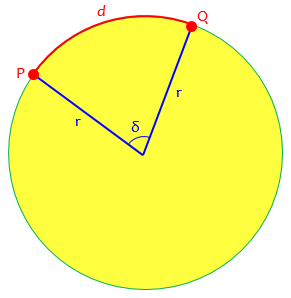### An example

Let’s check out the formula for Clarence’s example: San Francisco (N37 37′ 00″ latitude, W122 22′ 00″ longitude) and Paris (N48 44′ 00″ latitude, E02 23′ 00″ longitude), which turn out to be at San Francisco and Orly airports. Here $$(a_1,b_1) = (37.617°,-122.367°)$$ and $$(a_2,b_2) = (48.733°,2.383°)$$, and I’ll take $$r=3963\text{ miles}$$ as measured on the equator. Our formula gives $$\arccos\left[\cos(a_1)\cos(b_1)\cos(a_2)\cos(b_2)+\cos(a_1)\sin(b_1)\cos(a_2)\sin(b_2)+\sin(a_1)\sin(a_2)\right]\frac{2\pi r}{360}\\ = \arccos\left[\begin{matrix}\cos(37.617°)\cos(-122.367°)\cos(48.733°)\cos(2.383°)+\\ \cos(37.617°)\sin(-122.367°)\cos(48.733°)\sin(2.383°)+\\ \sin(37.617°)\sin(48.733°)\end{matrix}\right]\frac{\pi \cdot 3963}{180}\\ =\arccos\left[\begin{matrix}0.7921\cdot -0.5353\cdot 0.6596\cdot 0.99914+\\ 0.7921\cdot -0.8446\cdot 0.6596\cdot 0.04158+\\ 0.6104\cdot 0.7516\end{matrix}\right]\frac{3963\pi}{180}\\ =\arccos[0.16099]\frac{3963\pi}{180} =80.74°\frac{3963\pi}{180} =5584\text{ miles}$$

Google says it’s 5560 miles, which is close enough considering we don’t know where Google is measuring between, and the earth isn’t exactly spherical.

Two years later (probably as a result of comments from users), he added a note:

I thought I'd add a couple of remarks that may help some people use the above formula.  First, of all, you need to make sure your calculator or computer is using degrees, not radians, to figure out the Sine, Cosine, and ArcCosine functions.  If you're using a calculator or computer that uses radians, then use a different version of the formula:

Arccos[Cos[a1] Cos[b1] Cos[a2] Cos[b2] + Cos[a1] Sin[b1] Cos[a2] Sin[b2]     + Sin[a1] Sin[a2]] * r

I used a calculator in degree mode for my calculations above; I’d have to convert to radians to use Excel or other kinds of programming.

Also, keep in mind that these formulae don't take into account the squashed nature of the earth.  As you may know, the earth is kind of fat around the equator, as a result of the centrifugal force it gets from spinning on its axis.  So that will throw a little error into these calculations.  I've never tried to come up with a formula that takes the squashing into account, and I suspect it might be hard.

Such a formula has to take into account all the details of the actual shape of the earth, and is well beyond our scope. For an introduction, see here.

### Simplifying the cosine formula

Another four years later (1999) we got this question from Nat, which was added into the same page:

I saw Chris Michels' Latitude/Longitude Distance Calculation page at

http://jan.ucc.nau.edu/~cvm/latlongdist.html

which he says is based on the above answer. I recently needed to program a latitude, longitude, distance calculation and found a formula in the SAS tech page (as well as a few other places).  I think that your formula can be reduced to the following (and they give the same results):

A = LAT1, B = LONG1
C = LAT2, D = LONG2 (all converted to radians: degree/57.29577951)

IF A = C AND B = D THEN DISTANCE = 0;
ELSE

IF [SIN(A)SIN(C)+COS(A)COS(C)COS(B-D)] > 1 THEN DISTANCE =
3963.1*ARCOS; /* solved a prob I ran into.  I haven't fully
analyzed it yet */
ELSE

DISTANCE=3963.1*ARCOS[SIN(A)SIN(C)+COS(A)COS(C)COS(B-D)];

With more time I would solidify and show that it equals your formula.

Chris Michels’ formula is the same as ours using radians: $$r\arccos\left[\cos(a_1)\cos(b_1)\cos(a_2)\cos(b_2) + \cos(a_1)\sin(b_1)\cos(a_2)\sin(b_2) + \sin(a_1)\sin(a_2)\right]$$

Nat’s formula, in our terms and still using radians, is: $$r \arccos\left[\sin(a_1) \sin(a_2) + \cos(a_1) \cos(a_2) \cos(b_1-b_2)\right]$$

Using degrees, it is: $$\frac{\pi r}{180}\arccos\left[\sin(a_1) \sin(a_2) \sin(a_2) + \cos(a_1) \cos(a_2) \cos(b_1-b_2)\right]$$

Doctor Rick responded:

On our site, you can find several formulas, including yours. Chris Michels did not find the simplest formula in our archives. He did not find the most reliable formula, either; another, the Haversine formula, is supposed to be less prone to rounding errors under certain conditions.


The “simplest formula” is the next one we’ll be seeing, which was already available at that time (and identical to Nat’s); and the “most reliable” is the one we’ll see next week, which was explained by Doctor Rick in 2001.

You're correct that the formula he found can be simplified to yours. It's easy to start with yours and obtain his, by applying the angle-difference identity

cos(b-d) = cos(b)*cos(d) + sin(b)*sin(d)


Here is the work, which starts with a rather obvious factoring: $$r\arccos\left[\cos(a_1)\cos(b_1)\cos(a_2)\cos(b_2) + \cos(a_1)\sin(b_1)\cos(a_2)\sin(b_2) + \sin(a_1)\sin(a_2)\right]\\= r\arccos\left[\cos(a_1)\cos(a_2)(\cos(b_1)\cos(b_2) + \sin(b_1)\sin(b_2)) + \sin(a_1)\sin(a_2)\right]\\= r\arccos\left[\cos(a_1)\cos(a_2)(\cos(b_1-b_2)) + \sin(a_1)\sin(a_2)\right]$$

You can also follow through the derivation of the more complicated formula and modify it by rotating the cartesian coordinate system so that point 1 is in the x-z plane. Then the y coordinate of point 1 is 0, which causes terms to vanish. The x coordinate of point 2 is r*cos(a1)*cos(b2-b1), and the y coordinate of point 2 is r*cos(a1)*sin(b2-b1).

In other words, we can just rotate the globe to move the first point to the Greenwich meridian, so the longitude $$b_1$$ becomes zero, and the other becomes the difference of the two. Then the original formula becomes $$r\arccos\left[\cos(a_1)\cos(0)\cos(a_2)\cos(b_2-b_1) + \cos(a_1)\sin(0)\cos(a_2)\sin(b_2-b_1) + \sin(a_1)\sin(a_2)\right]\\= r\arccos\left[\cos(a_1)\cos(a_2)\cos(b_2-b_1) + \sin(a_1)\sin(a_2)\right]$$

### The example again

Let’s check this formula against the San Francisco to Paris data. We had San Francisco as $$(a_1,b_1) = (37.617°,-122.367°)$$ and Paris as $$(a_2,b_2) = (48.733°,2.383°)$$ So we get $$r\arccos\left[\sin(a_1) \sin(a_2) + \cos(a_1) \cos(a_2) \cos(b_1-b_2)]\\ = 3963 \arccos[\sin(37.617°) \sin(48.733°) + \cos(37.617°) \cos(48.733°) \cos(-122.367°-2.383°)\right]\\ = 3963 \arccos\left[0.6104\cdot 0.7516 + 0.7921\cdot 0.6596\cdot -0.5700\right]\\ = 3963 \arccos[0.16097]\\ = 3963\cdot\frac{\pi}{180}\cdot 80.737\\ = 5584\text{ miles}$$ Unsurprisingly, that’s what we got before. (Because I had my calculator set to degrees, I had to convert the arccos from degrees to radians at the end.)

## Direct derivation of the simpler form

A similar question arrived in 1996, before Nat’s question above, and presumably referring to the first (1995) answer we looked at:

Distance Between Two Points on the Earth

Dear Dr. Math,

I have found your article on calculating the distance between two points given latitude and longitude, but I can't seem to come up with the right answer. I don't think I'm using your equations correctly.

My latitude and longitude are in the form 40.266934, -74.204930 respectively, with negatives for South and West. Given two points in this form, how do I calculate the distance between them?

Thank you very much,

There are several possible mistakes Jonathan could have made; I struggled to get correct answers for my example, even though I knew when to use degrees or radians, and similar details! If this question came today, I would start by asking to see his work, so I could find the problem. But this was before that was easy to do.

Doctor Anthony replied, starting from scratch:

I will repeat the calculation here as I am not sure if it is one of my posts to which you are referring.

The calculation is done using the scalar product of two vectors to find the angle between those vectors. Let the vectors be OA and OB where A and B are the two points on the surface of the earth and O is the centre of the earth.

The scalar product gives OA*OB*cos(AOB)  = R^2*cos(AOB) where R = radius of the earth.  Having found angle AOB, the distance between the points is R*(AOB) with AOB in radians.

Here is our picture labeled his way, including the coordinate axes he’ll describe in a moment: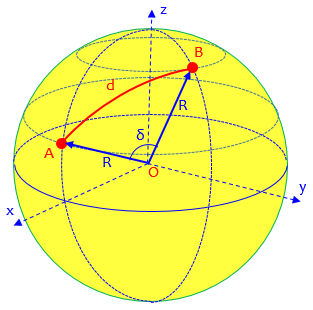To find the scalar product we need the coordinates of the two points.  Set up a three dimensional coordinate system with the x-axis in the longitudinal plane of OA and the xy plane containing the equator, the z-axis along the earth's axis.  With this system, the coordinates of A will be

Rcos(latA), 0, Rsin(latA)

and the coordinates of B will be

Rcos(latB)cos(lonB-lonA), Rcos(latB)sin(lonB-lonA), Rsin(latB)

Observe that he has rotated the coordinate system as described in the last comment in the previous answer.

The scalar product is given by

xA*xB + yA*yB + zA*zB = R^2cos(latA)cos(latB)cos(lonB-lonA)+ R^2sin(latA)sin(latB)

Dividing out R^2 will give cos(AOB)

cos(AOB) = cos(latA)cos(latB)cos(lonB-lonA) + sin(latA)sin(latB)

This gives AOB, and the great circle distance between A and B will be

R*(AOB)     with AOB in radians.

This is the same as our simplified cosine formula above: $$R\arccos\left[\cos(lat_1)\cos(lat_2)\cos(lon_2-lon_1) + \sin(lat_1)\sin(lat_2)\right]$$

### Another example

I will do an example, finding the distance between point A at 56 degrees west 33 degrees south, and point B at 12 degrees east and 40 degrees north.  [Note, I shall be taking east and south as negative.]

cos(AOB)= cos(-33)cos(40)cos(-12-56) + sin(-33)sin(40)
= cos(33)cos(40)cos(68) - sin(33)sin(40)
= -0.109417873

So AOB = 96.28175959 degrees

Finally to get the great circle distance between A and B we need the value of R, the radius of the earth. This is about 6371 km or 3959 miles.

In miles the distance between A and B is 6652.84 miles.

It doesn’t matter which directions you take as positive and negative, as long as you are consistent. He is using a slightly different radius for the earth, as many different values can be found depending on how it is measured.

Just for fun, I went to WolframAlpha and asked it “distance from 56 degrees west 33 degrees south, to 12 degrees east and 40 degrees north”. Here is its response: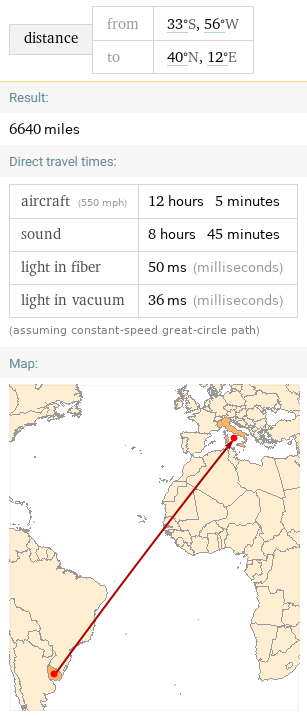There are several good reasons for the difference, including the non-sphericity of the earth.

## Same formula, different conventions

In 1997, we had seen a similar question:

Distance using Latitude and Longitude

Is there a simple formula for calculating distance using known latitude and longitude?

Doctor Rob answered, stating without proof essentially the same formula we just saw, but with different variables:

Yes.

Assume the Earth is a perfect sphere.  Let all angles be measured in degrees.

If the latitude is North, let phi = 90 - latitude. If the latitude is South, let phi = 90 + latitude. The North Pole has phi = 0, the South Pole has phi = 180, and 0 <= phi <= 180.

If the longitude is East, let theta = longitude. If the longitude is West, let theta = -longitude. Greenwich, England, has theta = 0, and -180 <= theta <= 180.

Let the angles for the two points be (phi1, theta1) and (phi2, theta2). Then compute

c = sin(phi1)*sin(phi2)*cos(theta1-theta2) + cos(phi1)*cos(phi2).

Then the shortest great circle distance between the two points is

d = R*Arccos(c)*Pi/180,

where R is the radius of the earth in miles, and the arccosine is taken between 0 and 180 degrees, inclusive.

His phi ($$\phi$$) and theta ($$\theta$$) are the standard angles in spherical coordinates as used by mathematicians.

Recall that our last formula was $$\arccos\left[\sin(a_1)\sin(a_2) +\cos(a_1)\cos(a_2)\cos(b_1-b_2)\right] r$$

This formula is instead $$\arccos\left[\sin(\phi_1)\sin(\phi_2)\cos(\theta_1-\theta_2) +\cos(\phi_1)\cos(\phi_2)\right] r$$

The answer from Dr. Rob to a student's question posting [see below] has an incorrect formula.

c = sin(phi1)*sin(phi2)*cos(theta1-theta2) + cos(phi1)*cos(phi2).

should be:

c = sin(phi1)*sin(phi2)+cos(theta1-theta2) * cos(phi1)*cos(phi2).

The difference appears to be the interchanging of an addition and a multiplication. A typo?

Doctor Rick replied,

Hi, Tom. Did you notice the way Dr. Rob defined phi? If phi is simply the latitude (+ for north, - for south latitude), then your formula is correct - it is the formula I use. But  Dr. Rob defined phi as 90 - lat for north latitudes (90 + lat for south latitudes). This definition switches sines and cosines, since sin(90-phi) = cos(phi) and cos(90-phi) = sin(phi).

Dr. Rob defined phi this way because it is the standard way that spherical coordinates are defined. Admittedly it's a little confusing to those who are used to working with latitudes, but then, those who are NOT accustomed to latitude math are more likely to ask the question!

Mistakes do sometimes make it into our archives, and we appreciate diligent readers who catch these errors. But I don't think we are wrong here. Keep looking! :-)

In fact, Doctor Rob’s version amounts to the Spherical Law of Cosines for Sides, as applied to this spherical triangle: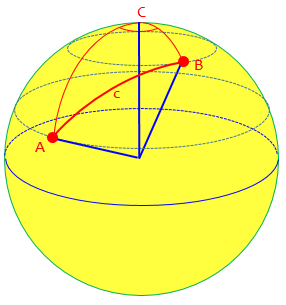### 1 thought on “Distances on Earth 1: The Cosine Formula”

This site uses Akismet to reduce spam. Learn how your comment data is processed.